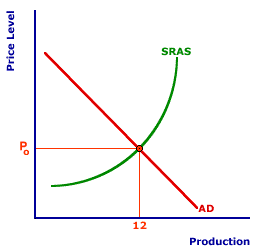Tuesday  January 31, 2023
 AmosWEB means Economics with a Touch of Whimsy!AS-AD MODEL: An economic model relating the price level and real production that is used to analyze business cycles, gross domestic product, unemployment, inflation, stabilization policies, and related macroeconomic phenomena. The AS-AD model, inspired by the standard market model, captures the interaction between aggregate demand (the buyers) and short-run and long-run aggregate supply (the sellers).MULTIPLIER, AGGREGATE MARKET:

The magnitude of the multiplier impact of an autonomous expenditure change is smaller in the aggregate market analysis, due to a change in the price level, than in the standard Keynesian cross analysis, which assumes a constant price level. The change in the price level triggers an opposite change in aggregate expenditures to that of the initial autonomous expenditure change, thus reducing the magnitude of the multiplier impact.
When evaluated using the aggregate market, the standard Keynesian multiplier impact is dampened by price level changes. Changes in the price level trigger the real-balance effect, interest-rate effect, and net-exports effect, which cause a movement along the aggregate demand curve and subsequent changes in aggregate expenditures. These aggregate expenditures change in the opposite direction of the initial change in the autonomous expenditures that set the multiplier process in motion.

Standard Keynesian analysis is based on the assumption that the price level is constant or an exogenous variable of the model. Aggregate market analysis, in contrast, includes the price level as an endogenous variable. In Keynesian analysis aggregate production is assumed to rise or fall to match any changes in aggregate expenditures. In aggregate market analysis aggregate production is more realistically subject to constraints which are reflected in a price level changes.

### The Aggregate Market

The Aggregate MarketAn overview of the aggregate market is in order. The exhibit to the right presents the standard short-run aggregate market analysis. The negatively-sloped aggregate demand curve is labeled AD and the positively-sloped short-run aggregate supply curve is labeled SRAS. The intersection of the two curves indicates equilibrium at price level Po and aggregate production of \$12 trillion.

Key to this present discussion, any shock to the aggregate market, especially caused by a shift of the aggregate demand curve, results in a change in the price level. Moreover, this price level change will then cause a movement along the aggregate demand curve as equilibrium is restored.

With this in mind, suppose that there is an increase in aggregate demand, such as that caused by a \$1 trillion increase in investment expenditures. This, of course, causes a rightward shift of the aggregate demand curve and results in a new equilibrium. Click the [AD Increase] button to illustrate this shift.

The new equilibrium is achieved at a higher price level, P1, and a larger level of aggregate production, \$13 trillion, given by the intersection of the new aggregate demand curve and the original short-run aggregate supply curve.

### A Keynesian Comparison

How does this adjustment compare to the standard Keynesian multiplier process? To answer this question, let's dissect this adjustment.
• Consider the rightward shift of the aggregate demand curve. The standard Keynesian multiplier analysis assumes that the price level remains unchanged. As such, the initial horizontal shift of the aggregate demand curve indicates the Keynesian multiplier. Click the [Keynesian Multiplier] button to illustrate.

• The horizontal shift of the aggregate demand curve, measured at the original price level, Po, is the change in aggregate production resulting from the Keynesian multiplier. This is indicated by the movement from point A to point C. This level of aggregate production is \$16 trillion, a \$4 trillion increase resulting from a \$1 trillion investment injection and a multiplier of 4.

• However, the aggregate market analysis indicates that the price level does not remain constant at Po, but instead rises to P1. This increase in the price level leads to the real-balance, interest-rate, and net-export effects, which cause a movement along the aggregate demand curve from point C to point B.

• The higher price level, as such, dampens the original Keynesian multiplier process. Rather than a multiplier of 4, which triggers the \$4 trillion change that achieves \$16 trillion in aggregate production, the price-adjusted multiplier is only 1 in this case. At the short-run equilibrium intersection point B, the change in aggregate production is only \$1 trillion, and the equilibrium level of aggregate production is \$13 trillion.
The key conclusion from this aggregate market analysis is that the multiplier process depends on the price level and the supply side of the economy. When the price level changes, the full Keynesian multiplier is not realized.

This conclusion can be taken a step farther with the long-run aggregate market. In the long run, aggregate production remains constant at full employment. As such, there is no change in aggregate production, regardless of the change in aggregate expenditures. In this case, the multiplier is zero, there is no multiplier. Period.

 <= MULTIPLIER MULTIPLIER, INJECTIONS-LEAKAGES MODEL =>Recommended Citation:

MULTIPLIER, AGGREGATE MARKET, AmosWEB Encyclonomic WEB*pedia, http://www.AmosWEB.com, AmosWEB LLC, 2000-2023. [Accessed: January 31, 2023].

Check Out These Related Terms...

Or For A Little Background...

And For Further Study...
Search Again?BLACK DISMALAPOD[What's This?] Today, you are likely to spend a great deal of time searching for a specialty store wanting to buy either a lazy Susan for you dining room table or a set of serrated steak knives, with durable plastic handles. Be on the lookout for empty parking spaces that appear to be near the entrance to a store.Your Complete ScopeThe 1909 Lincoln penny was the first U.S. coin with the likeness of a U.S. President."To understand a man, you must know his memories. The same is true of a nation."-- Anthony Quayle, ActorTGETokyo Grain Exchange (Japan)A PEDestrian's Guide Xtra CreditTell us what you think about AmosWEB. Like what you see? Have suggestions for improvements? Let us know. Click the User Feedback link.| | | | | | | | | | |
| | | |

Thanks for visiting AmosWEB